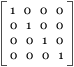2. 3D Math Basics The following is a short introduction into the world of 3D math. Note that this is not a math tutorial, it only covers the basic stuff you need to work with 3D objects. In addition, there's a number of links pointing you to more 3D knowledge on the web. 2.1 Points & Vectors A point in the three dimensional space can be defined as P(x, y, z). Creating a new point in 3dhtml is just as simple as that: ```var myPoint = new Point3D(x, y, z); ``` The Point is internal stored as homogeneous coordinates, i.e. a four element point with an additional coordinate w. We treat the point as 1x4 matrix called a vector. 2.2 Matrices What is the matrix? Well, a matrix is defined as a rectangular array of numbers. (cough) In 3D math we use 1x4 matrices to represent a point and 4x4 matrices to transform these points.4x4 Unit matrix We will need matrix multiplication so it's the only matrix math we are looking at: ```a1 a2 b1 b2 (a1 * b1 + a2 * b3) (a1 * b2 + a2 * b4) a3 a4 x b3 b4 = (a3 * b1 + a4 * b3) (a3 * b2 + a4 * b4) ``` Okay, that was just a 2x2 matrix multiplication, we have to handle with 4x4 and that's exactly the reason why 3dhtml tries to conceal that stuff. 2.3 Transformations We can move points in the three dimensional space to new positions by adding values to the coordinates. To translate the point P(2, 0, 1) to the new position (3, 2, 1) we have to add (1, 2, 0). Other transformations are scaling and rotating. These are more complex and result in a matrix multiplication. Since we express the Point3D in homogeneous coordinates we can treat all transformations simply as multiplications: Translation with (dx, dy, dz) ``` 1 0 0 dx 0 1 0 dy 0 0 1 dz 0 0 0 1 ``` Scaling with scale factors (sx, sy, sz) ```sx 0 0 0 0 sy 0 0 0 0 sz 0 0 0 0 1 ``` Rotation with phi (φ) degrees around the x axis ```1 0 0 0 0 cos(phi) -sin(phi) 0 0 sin(phi) cos(phi) 0 0 0 0 1 ``` That was just to get a rough impression of how the transformation matrices look like. Use these transformations as shown in 4.1 Cube3D # Transformation. 2.4 Matrix Composition Instead of transform a point multiple compose the transformations matrices and render the new coordinates only once. Suppose we want to translate and scale two points the same way we have to process four transformations. By composing these transformations we reduce the number of transformations to process to two (and one matrix multiplication). Mostly you will use matrix composition indirectly. Just call the transformation methods. In the following code example you'll get a matrix to rotate and scale a model. ```var myMatrix = new Matrix(); myMatrix.rotateX(0.05); myMatrix.scale(2, 2, 2); ``` If you want to combine two matrices use `myMatrix.compose(otherMatrix)`. For example you want to transform one model depending on mouse interaction and the other model shall be transformed by mouse movements, too but also by a static bumping (size-varying) matrix. So use `heartBeatMatrix.compose(mouseMatrix)` and use the (now composed) heartBeatMatrix to transform the second object. Links: Beginner: The Mathematics of 3D Graphics Really simple beginner tutorial. With an introduction into directions, vectors among others. Matrix MathA useful tutorial. 3D Transformations Good and detailed tutorial. Be aware that because of the different coordinate system the matrices are flipped. 3D dictionary Huge dictionary focused on 3D computer graphics. Some entries with images.